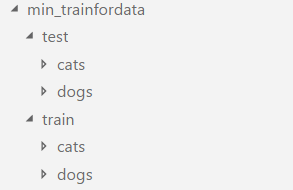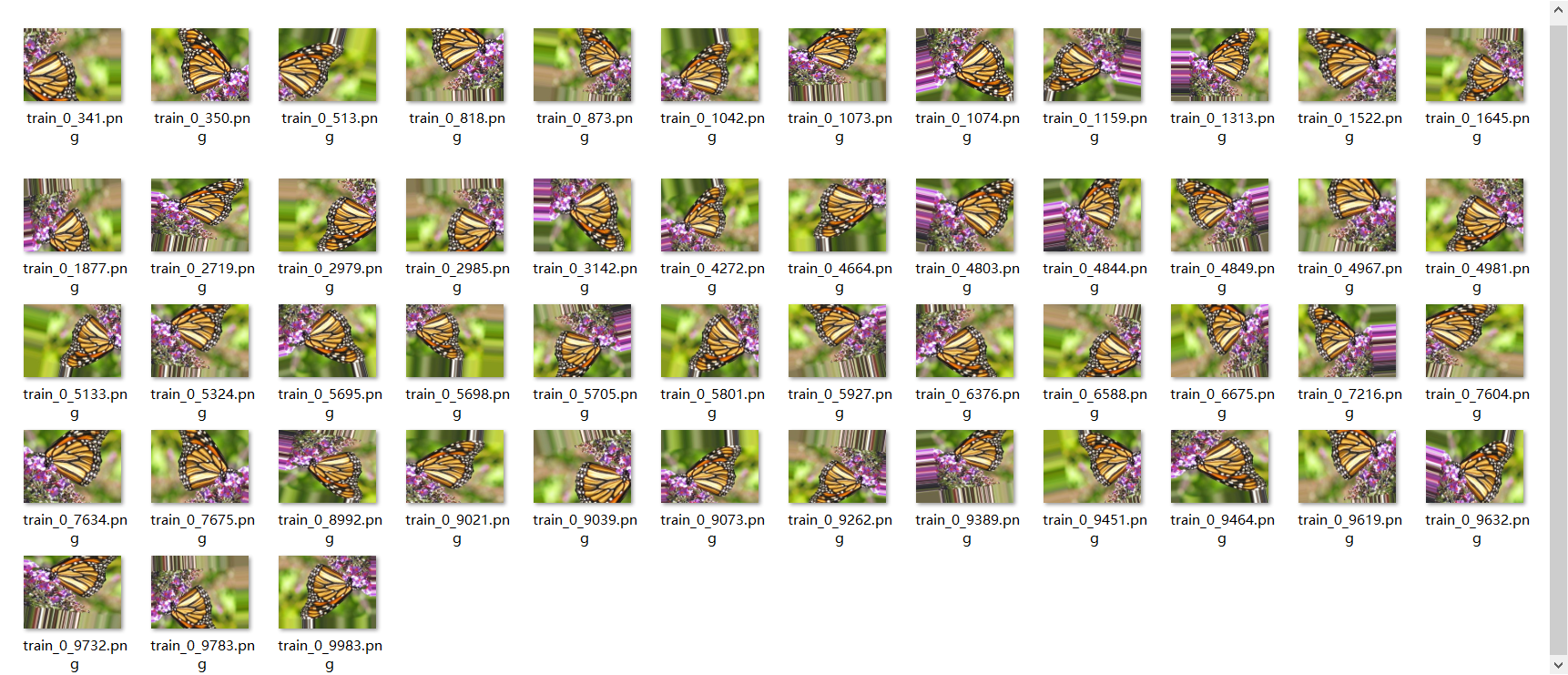2019-02-08 23:20:33 weixin_39965184 阅读数 242
• ###### 人工智能框架实战精讲：Keras项目

Keras项目实战课程从实战的角度出发，基于真实数据集与实际业务需求，从零开始讲解如何进行数据处理，模型训练与调优，最后进行测试与结果展示分析。全程实战操作，以最接地气的方式详解每一步流程与解决方案。课程结合当下深度学习热门领域，以计算机视觉与自然语言处理为核心讲解各大网络的应用于实战方法，适合快速入门与进阶提升。 任务作业： 1.基于Keras构建VGG网络模型 2.加载与预处理细胞图像数据 3.构建完成分类模型并进行测试识别 （注意： 作业需写在CSDN博客中，请把作业链接贴在评论区，老师会定期逐个批改~~）

1452 人正在学习 去看看 唐宇迪

## 1.keras训练minst数据集

1. 环境keras，jupyter notebook
2. 加载keras模块
``````from __future__ import print_function
import numpy as np
np.random.seed(1337)

from keras.datasets import mnist
from keras.models import Sequential
from keras.layers.core import Dense,Dropout,Activation
from keras.utils import np_utils
``````
1. 如需绘制模型请加载plot
``````from keras.utils.vis_utils import plot_model
``````
1. 变量初始化
``````batch_size=128
nb_classes=10
nb_epoch=20
``````
1. 定义加载数据集函数（keras自带数据集下载不了，被防火墙挡着）
``````def load_data(path='mnist.npz'):
#Arguments
path:path where to cache the dataset locally
#Returns
Tuple of Numpy arrays:`(x_train,y_train),(x_text,y_text)`

"""
path='.\mnist.npz'
x_train,y_train=f['x_train'],f['y_train']
x_test,y_test=f['x_test'],f['y_test']
f.close()
return (x_train,y_train),(x_test,y_test)
``````
1. 数据准备
``````(X_train, y_train), (X_test, y_test) =load_data()
X_train = X_train.reshape(60000, 784)
X_test = X_test.reshape(10000, 784)
X_train = X_train.astype('float32')
X_test = X_test.astype('float32')
X_train /= 255
X_test /= 255
print(X_train.shape, 'train samples')
print(X_test.shape, 'test samples')
``````
1. 转换类标号
``````# convert class vectors to binary class matrices
Y_train = np_utils.to_categorical(y_train, nb_classes)
Y_test = np_utils.to_categorical(y_test, nb_classes)
``````
1. 建立模型
`#使用Sequential() model = Sequential() model.add(Dense(512, input_shape=(784,))) model.add(Activation('relu')) model.add(Dropout(0.2)) model.add(Dense(512)) model.add(Activation('relu')) model.add(Dropout(0.2)) model.add(Dense(10)) model.add(Activation('softmax'))`
2. 打印模型
``````model.summary()
``````
1. 绘制模型结构图，并保存成图片
``````plot_model(model,to_file='model.png')
``````
1. 训练与评估
`#编译模型 model.compile(loss='categorical_crossentropy', optimizer=RMSprop(), metrics=['accuracy'])`

2. #迭代训练

``````history = model.fit(X_train, Y_train,
batch_size=batch_size, nb_epoch=nb_epoch,
verbose=1, validation_data=(X_test, Y_test))`
``````
1. 模型评估
``````score = model.evaluate(X_test, Y_test, verbose=0)
print('Test score:', score)
print('Test accuracy:', score)
``````
1. 模型保存
``````model.save('mnist-mpl.h5')
``````

keras 产品 大数据平台 相关内容

2018-04-15 16:58:08 nima1994 阅读数 4834
• ###### 人工智能框架实战精讲：Keras项目

Keras项目实战课程从实战的角度出发，基于真实数据集与实际业务需求，从零开始讲解如何进行数据处理，模型训练与调优，最后进行测试与结果展示分析。全程实战操作，以最接地气的方式详解每一步流程与解决方案。课程结合当下深度学习热门领域，以计算机视觉与自然语言处理为核心讲解各大网络的应用于实战方法，适合快速入门与进阶提升。 任务作业： 1.基于Keras构建VGG网络模型 2.加载与预处理细胞图像数据 3.构建完成分类模型并进行测试识别 （注意： 作业需写在CSDN博客中，请把作业链接贴在评论区，老师会定期逐个批改~~）

1452 人正在学习 去看看 唐宇迪1 .如下代码，获取所有猫狗文件路径，可以看出共分别有12500，12500猫狗图片。

``````import os

train='E:/MLdata/kaggle_Dogsvs.Cats/train/'

dogs=[train+i for i in os.listdir(train) if 'dog' in i]

cats=[train+i for i in os.listdir(train) if 'cat' in i]

print(len(dogs),len(cats))
``````

12500 12500

2 . 如下代码，将训练用到2000张图片，测试的1000张图片放置指定文件夹。

``````import os
import shutil

def createDir(path):
if not os.path.exists(path):
try:
os.makedirs(path)
except:
print("创建文件夹失败")
exit(1)

path="E:/MLdata/kaggle_Dogsvs.Cats/min_trainfordata/"

createDir(path+"train/dogs")
createDir(path+"train/cats")
createDir(path+"test/dogs")
createDir(path+"test/cats")

for dog,cat in list(zip(dp.dogs,dp.cats))[:1000]:
shutil.copyfile(dog,path+"train/dogs/"+os.path.basename(dog))
print(os.path.basename(dog)+"操作成功")
shutil.copyfile(cat, path + "train/cats/" + os.path.basename(cat))
print(os.path.basename(cat) + "操作成功")
for dog, cat in list(zip(dp.dogs, dp.cats))[1000:1500]:
shutil.copyfile(dog, path + "test/dogs/" + os.path.basename(dog))
print(os.path.basename(dog) + "操作成功")
shutil.copyfile(cat, path + "test/cats/" + os.path.basename(cat))
print(os.path.basename(cat) + "操作成功")

``````

keras 产品 大数据平台 相关内容

2019-07-27 12:40:31 zhouchen1998 阅读数 267
• ###### 人工智能框架实战精讲：Keras项目

Keras项目实战课程从实战的角度出发，基于真实数据集与实际业务需求，从零开始讲解如何进行数据处理，模型训练与调优，最后进行测试与结果展示分析。全程实战操作，以最接地气的方式详解每一步流程与解决方案。课程结合当下深度学习热门领域，以计算机视觉与自然语言处理为核心讲解各大网络的应用于实战方法，适合快速入门与进阶提升。 任务作业： 1.基于Keras构建VGG网络模型 2.加载与预处理细胞图像数据 3.构建完成分类模型并进行测试识别 （注意： 作业需写在CSDN博客中，请把作业链接贴在评论区，老师会定期逐个批改~~）

1452 人正在学习 去看看 唐宇迪

# 数据增广

## 简介

• 在实际的深度学习项目中，数据集的需求是非常大的，强大的模型包含更多的参数，训练这些参数需要大量的数据；大量的数据训练使得模型的泛化能力变强，一定程度上克制过拟合的出现。
• 数据增广是对数据集进行倍增的有效手段，首次成功应用于AlexNet取得巨大的效果。对图片增广其主要含义为对原来的图片进行翻转（水平翻转和垂直翻转）、扭曲、变形、拉伸、填充、换色、裁减等手段产生新的图片，该图片近似于原图分布且对模型而言是全新的图片，从而达到获取更多训练数据且有标注（标注与原图一致或可以通过变换得到）的目的。
• Keras作为一个成熟的方便的深度学习框架提供了很高效的图片数据增广的API，本项目将逐一演示其作用。

## 原则

• 数据增广的手段是以任务为驱动的，是对任务有利的而不是有害的。
• 不恰当的数据增广会干扰模型的训练，而不会提高模型效果。（如将手写数据垂直翻转没有意义，没有人的手写字是倒置的且会使模型难以拟合。）
• 不是所有时候数据增广都是有效果的，有时候即使正确的增广未必会达到预期的效果，这是模型的问题。

## 数据增广

• 说明

• Keras要求在创建生成器的时候就指定增广方式，所以对于训练数据和测试数据必须创建不同的生成器（要求训练数据一般情况下是不需要增广的）。**注意，生成器只是生成器，要想生成数据需要调用生成器的flow方法或者flow_*方法才能得到数据集，调用flow得到的生成器才是fit_generator方法需要的生成器。
• 参数说明
• ``````  from keras.preprocessing.image import ImageDataGenerator
import keras.backend as K

train_gen = ImageDataGenerator(
featurewise_center=False,
samplewise_center=False,
featurewise_std_normalization=False,
samplewise_std_normalization=False,
rescale=1/255.,
zca_whitening=False,
zca_epsilon=1e-6,
rotation_range=0.,
width_shift_range=0.,
height_shift_range=0.,
shear_range=0.,
zoom_range=0.,
channel_shift_range=0.,
fill_mode='nearest',
cval=0.,
horizontal_flip=False,
vertical_flip=False,
preprocessing_function=None,
data_format=K.image_data_format())
``````
• 说明
• featurewise_center：布尔型，输入数据集去中心化（均值为0）。
• samplewise_center：布尔型，输入数据每个样本均值为0。
• featurewise_std_normalization：布尔型，将输入样本除以数据集的标准差以完成标准化。
• samplewise_std_normalization：布尔型，将输入样本除以样本自身的标准差以完成标准化。
• rescale：数值型，重放缩因子，默认为None。如果为None或0则不进行放缩,否则会将该放缩因子乘到样本数据上(在应用任何其他变换之前），一般设定为1/255.用于图片归一化。
• zca_whitening：布尔型，对输入数据进行ZCA白化。
• zca_epsilon: 数值型，ZCA白化使用的eposilon，默认1e-6。
• rotation_range：整型，增广时图片随机转动的角度，取值为0-180。
• width_shift_range：数值型，图片宽度的某个比例值，增广时图片水平偏移的幅度。
• height_shift_range：数值型，图片高度的某个比例值，增广时图片竖直偏移的幅度。
• shear_range：数值型，剪切强度（逆时针方向的剪切变换角度）。
• zoom_range：数值型或[low, high]的列表，随机缩放的幅度，数值型表示[low, high]=[1-zoom_range, 1+zoom_range]。
• channel_shift_range：数值型，通道偏移的幅度。
• fill_mode：‘constant’,‘nearest’,'reflect’或’wrap’取值之一，当增广越出边界按该值指定的方法处理。
• cval：数值型，当fill_mode为’constant’时，越界点的填充值。
• horizontal_flip：布尔型，是否随机进行水平翻转。
• vertical_flip：布尔型，是否随机进行竖直翻转。
• preprocessing_function：将被应用于每个输入的函数。该函数将在图片缩放和数据增广之后运行。该函数接受一个参数，为一张图片（ndarray），并且输出一个具有相同shape的ndarray。
• data_format：'channel_first’或’channel_last’之一，代表图像的通道维的位置。numpy类型图片通道维在最后，如(224,224, 3)。
• flow方法参数说明
• ``````  train_generator = train_gen.flow(
X,
y,
batch_size=1,
shuffle=True,
seed=None,
save_to_dir=None,
save_prefix='',
save_format='png')
``````
• 说明
• flow方法会死循环地返回一批随机增广后数据及其标签（在y不为None时）。
• X：样本数据，四维数据。黑白图像的channel轴的值为1，彩色图像为3。
• y：与X第一维数值相同的标签数据。
• batch_size：批尺寸大小，默认为32。
• shuffle：是否随机打乱数据，默认为True。
• save_to_dir：默认为None，字符串类型，图片存储目录，该参数能让你将增广后的图片保存本地。
• save_prefix：字符串类型，保存增广后图片时使用的前缀（如train）, 仅当设置了save_to_dir时生效。
• save_format：‘png’或’jpeg’之一，指定保存图片的数据格式，默认’jpeg’。
• seed：随机数种子，保证复现。
• 增广

• 代码
• ``````  train_gen = ImageDataGenerator(
featurewise_center=False,
samplewise_center=False,
featurewise_std_normalization=False,
samplewise_std_normalization=False,
rescale=1/255.,
zca_whitening=False,
zca_epsilon=1e-6,
rotation_range=15,
width_shift_range=0.2,
height_shift_range=0.2,
shear_range=0.2,
zoom_range=0.2,
channel_shift_range=0.,
fill_mode='nearest',
cval=0.,
horizontal_flip=True,
vertical_flip=True,
preprocessing_function=None,
data_format=K.image_data_format())

train_generator = train_gen.flow(
X,
y,
batch_size=1,
shuffle=True,
seed=None,
save_to_dir='gene',
save_prefix='train',
save_format='png')

index = 0
for (batch_x, batch_y) in train_generator:
index += 1
if index > 50:
break
``````
• 结果
•• 可以看到，每一批都有概率进行了随机增广。

## 补充说明

• 本项目重点是数据增广，数据的多种读入方式没有过多提及。
• 具体代码上传至我的Github，欢迎Star或者Fork。
• 博客同步至个人博客网站，欢迎查看。

keras 产品 大数据平台 相关内容

2019-12-25 20:51:55 Andrew_SJ 阅读数 20
• ###### 人工智能框架实战精讲：Keras项目

Keras项目实战课程从实战的角度出发，基于真实数据集与实际业务需求，从零开始讲解如何进行数据处理，模型训练与调优，最后进行测试与结果展示分析。全程实战操作，以最接地气的方式详解每一步流程与解决方案。课程结合当下深度学习热门领域，以计算机视觉与自然语言处理为核心讲解各大网络的应用于实战方法，适合快速入门与进阶提升。 任务作业： 1.基于Keras构建VGG网络模型 2.加载与预处理细胞图像数据 3.构建完成分类模型并进行测试识别 （注意： 作业需写在CSDN博客中，请把作业链接贴在评论区，老师会定期逐个批改~~）

1452 人正在学习 去看看 唐宇迪

Keras下载的数据集在以下目录中：
win10
C:\Users\user_name.keras\datasets

boston_housing.npz
imdb.npz
mnist.npz

keras 产品 大数据平台 相关内容

2018-05-09 10:28:53 weixin_41770169 阅读数 5287
• ###### 人工智能框架实战精讲：Keras项目

Keras项目实战课程从实战的角度出发，基于真实数据集与实际业务需求，从零开始讲解如何进行数据处理，模型训练与调优，最后进行测试与结果展示分析。全程实战操作，以最接地气的方式详解每一步流程与解决方案。课程结合当下深度学习热门领域，以计算机视觉与自然语言处理为核心讲解各大网络的应用于实战方法，适合快速入门与进阶提升。 任务作业： 1.基于Keras构建VGG网络模型 2.加载与预处理细胞图像数据 3.构建完成分类模型并进行测试识别 （注意： 作业需写在CSDN博客中，请把作业链接贴在评论区，老师会定期逐个批改~~）

1452 人正在学习 去看看 唐宇迪

keras数据集主要有以下7种（可从keras官方文档阅读：https://keras.io/datasets/），对其中部分数据集我进行了学习和实践，并写了笔记。

1、CIFAR10小图像

``````from keras.datasets import cifar10

(x_train, y_train), (x_test, y_test) = cifar10.load_data()``````
• x_train, x_test: 参数规格分别为(50000, 3, 32, 32)和(10000, 3, 32, 32)
• y_train, y_test: 标签取值范围 (0-9)，shape (50000)和(10000)

2、CIFAR100小图像

``````from keras.datasets import cifar100

(x_train, y_train), (x_test, y_test) = cifar100.load_data(label_mode='fine')``````
• x_train, x_test: 参数规格分别为(50000, 3, 32, 32)和(10000, 3, 32, 32)
• y_train, y_test: 标签取值范围 (0-99)，shape (50000)和(10000)

3、IMDB电影影评情感分类

``````from keras.datasets import imdb

(x_train, y_train), (x_test, y_test) = imdb.load_data(path="imdb.npz",
num_words=None,
skip_top=0,
maxlen=None,
seed=113,
start_char=1,
oov_char=2,
index_from=3)``````
• x_train, x_test: 整数下标，如果num_words给定，则最大下标为num_words-1。
• y_train, y_test: 标签(1 or 0).

• path：数据存放位置，如果本地没有，会从网络下载
• num_words ：要考虑的最常见的单词。如果你不想考虑一些很晦涩的词汇，比如“Ultracrepidarian”，这会很有用。
• skip_top ：忽略的热门词汇。如果你不想考虑最常见的词，这会很有用。比如，单词“the”将不会在评论中添加任何信息，所以我们可以通过将`skip_top` 设置为 2 或更高来跳过它。
• maxlen：序列最大长度，如果有超过maxlen的序列，会进行截断。None表示不限制长度。
• seed：数据再生的种子
• start_char：序列开始的标记
• oov_char：被去掉的单词（比如因为num_words的限制，或者skip_top的限制）就用这个字符代替
• index_from：找到当前索引和大于当前索引的词

4、路透社新闻专线主题分类

``````from keras.datasets import reuters

(x_train, y_train), (x_test, y_test) = reuters.load_data(path="reuters.npz",
num_words=None,
skip_top=0,
maxlen=None,
test_split=0.2,
seed=113,
start_char=1,
oov_char=2,
index_from=3)```test_split=0.2,
seed=113,
start_char=1,
oov_char=2,
index_from=3)```

5、手写数字MNIST数据集

``````from keras.datasets import mnist

(x_train, y_train), (x_test, y_test) = mnist.load_data()``````
• x_train, x_test: 参数规格分别为(60000, 28, 28)和(10000, 28, 28)。
• y_train, y_test: 数字标签(0-9)，参数规格分别为(60000,)和(10000,)

6、时尚元素MNIST数据库

``````from keras.datasets import fashion_mnist

(x_train, y_train), (x_test, y_test) = fashion_mnist.load_data()``````
• x_train, x_test: 参数规格分别为(60000, 28, 28)和(10000, 28, 28)。
• y_train, y_test: 数字标签(0-9)，参数规格分别为(60000,)和(10000,)

7、波斯顿房价回归数据集

1970年代，波斯顿周边地区的房价。

``````from keras.datasets import boston_housing

(x_train, y_train), (x_test, y_test) = boston_housing.load_data()``````

keras 产品 大数据平台 相关内容